Subsections

# Integration Topics

## For Exercise 1 .....

In Sections 5.8 and 8.1 you are asked to find antiderivatives by substitution. This is really a change of variables'' technique used to better see how the integrand can be looked at as the derivative of some function. Maple has a command called changevar that can be used to practice substitution. This command has three arguments. In the first argument the quantity to be replaced is followed by an equals sign which is followed by what will be substituted. The second argument tells where the substitution will be made and the third argument gives the variable in terms of which the changed expression will be given. Note that when an integral in x is rewritten with changevar, the dx must also be changed, and in a definite integral, the limits of integration also need to the changed. Since changevar is in the student package, that package must be loaded before using changevar.

Here is an indefinite integral example of the use of changevar. We use Int instead of int because we do not want the integral evaluated immediately; we want to be able to observe the substitution process. After we have done that, we use value to get the answer. Then we use changevar one more time in order to express the answer in terms of the original variable of the problem. Keep in mind that the aim in doing a substitution is to simplify the integral to some pattern with which we can readily deal.

  > with(student):

  > g:=x->x*sqrt(1+x^2);

  > I1:=Int(g(x),x);

  > changevar(1+x^2=u,I1,u);

  > value();

  > changevar(u=1+x^2,'',x);

When you run these commands, notice that the integral is much simplified after the first change of variables. By the way, can you explain why the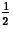appears in the output at that time?

Next we look at the commands for a definite integral.

  > I2:=Int(g(x),x=2..3);

  > changevar(1+x^2=t,I2,t);

  > value();


In this case we did not have to, in rewriting the integral, switch back to x after integrating because the limits of integration were switched from limits on x to limits on t. Make sure you understand how the limits on t were obtained.

## For Exercise 2 .....

You will find the background you need in a section of the text we have covered. Make sure that the answer you give is a reasonable one.

## For Exercise 3 .....

Here is a reminder that should be useful in this exercise. Let us say we need to find the roots of a polynomial function h(x). Of course, this can be done with fsolve. However if you name the output of fsolve, then you can have Maple give you each of the individual roots for further computation. For instance if

  > root:=fsolve(h(x)=0,x);

then root is the value of the first listed root root is the value of the second listed root, etc.

## For Exercise 4 .....

Volumes of solids of revolution are often found by using the disk/washer method. (See Section 6.2 of the text.) The Maple CalcP package has three commands that can help you in your study of this method. The command revolve gives a picture of the solid of revolution being considered. The LeftDisk command uses a regular partition with x*i chosen to be the left hand endpoint of the i-th subinterval, to give a picture of the approximating pieces, i.e. the disks or washers. For both of these commands the picture is 3-dimensional. You can click and drag on it in order to view it from different angles. Details of this will be discussed in lab.

The third command is LeftInt. This evaluates the Riemann sum associated with the picture given by LeftDisk. Note that the third argument in each of these specifies the numbers of subintervals.

Running the following code will help you see how these commands work

  > with(CalcP):

  > f:=x->x^2+1;

  > plot(f(x),x=-2..2);

  > revolve(f(x),x=-2..2);

  > revolve(f(x),x=-2..2,y=-2);

  > revolve({5,f(x)},x=-2..2);

  > LeftDisk(f(x),x=-2..2,5);

  > LeftDisk(f(x),x=-2..2,10);

  > LeftInt(f(x),x=-2..2,5);

  > LeftInt(f(x),x=-2..2,10);

  > Pi*int((f(x))^2,x=-2..2);

  > evalf();

The fifth line shows how to revolve the given area about a horizontal line other than the x-axis. Line 6 pertains to revolving the area between two curves; hence the washer method is linked to this volume. Reposition the picture so that you can look through the hole in the center of the solid. The second last line uses the formula developed in the text to find the volume of our solid of revolution. Since, for purposes of illustration, we have used a very simple f(x), the volume can be found exactly. In practice, this will not always be the case.

## Exercises

1.
Use a substitution to help evaluate the following integrals. Follow the patterns given in the background section. Use a substitution that results in an integral you could easily evaluate by hand. When you use your substitution, a constant factor shows up in the transformed integral. Explain where it comes from.
(a)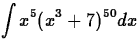(b)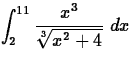2.
Consideron the interval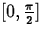. Use Maple to find the c whose existence is guaranteed by the Mean Value Theorem for Integrals. Also, give the mean value of the function on the given interval. Make sure your answers are reasonable.

3.
Find the total units of area enclosed by the graphs of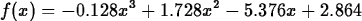and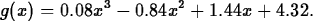What special care must be taken in this problem? What is the situation that complicates this problem? Explain.

4.
Solids of revolution.
(a)
Run the code given in the background section. Reposition each picture so that it looks 3-dimensional. In particular, display the hole'' mentioned in the background.
(b)
Given the functions f(x) = -x2 + 5x + 3 and g(x) = x2, consider the volume of the solid of revolution formed when the area enclosed by the two functions is rotated about the x-axis.
i.
Use revolve to picture the solid and LeftDisk with n=10 to picture the approximating pieces. Show good'' matching views of both pictures.
ii.
Set up and evaluate the integral that gives the volume.
iii.
Use LeftInt with n = 100 to estimate the volume. Is the number you get here close enough to the number you got in part ii) for you to feel confident that you handled both parts correctly?
(c)
Consider the two commands given below. Run them. They give approximating pieces for two different solids. For each of them, set up and evaluate the integral that gives the volume in question. In your words, describe the area being rotated. It would be a good idea to use LeftInt to see if your results seem reasonable.

  > LeftDisk(x^2,x=0..3,6,y=10);

  > LeftDisk({x^2,0},x=0..3,6,y=10);Next: About this document ... Up: Labs and Projects for Previous: Labs and Projects for

Christine Marie Bonini
4/8/1999